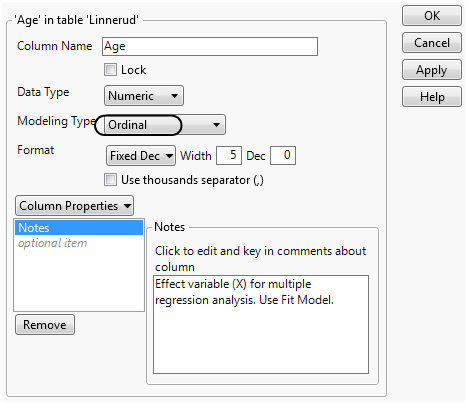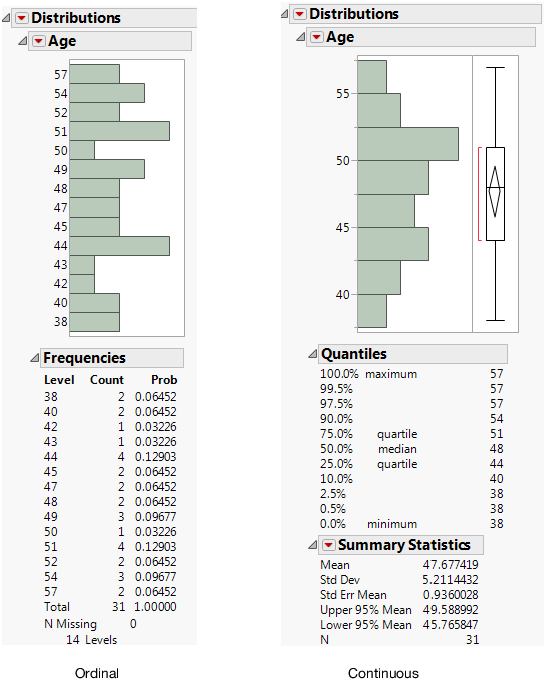Publication date: 05/24/2021

## Change the Modeling Type

To treat a variable differently, change the modeling type. For example, in Figure 5.4, the modeling type for Age is ordinal. Remember that for an ordinal variable, JMP calculates frequency counts. Suppose that you wanted to find the average age instead of frequency counts. Change the modeling type to continuous, which shows the mean age.

1. Double-click the Age column heading. The Column Info window appears.

2. Change the Modeling Type to Continuous.

Figure 5.5 Column Info Window3. Click OK.

4. Repeat the steps in the example (see Example of Viewing Modeling Type Results) to create the distribution. Figure 5.6 shows the distribution results when Age is ordinal and continuous.

Figure 5.6 Different Modeling Types for AgeWhen age is ordinal, you can see the frequency counts for each age. For example, age 48 appears two times. When age is continuous, you can find the mean age, which is nearly 48 (47.677)